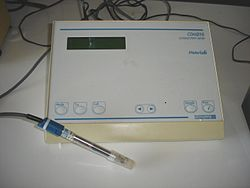# Electrical conductivity meter

﻿
Electrical conductivity meterAn electrical conductivity meter.

An electrical conductivity meter (EC meter) measures the electrical conductivity in a solution. Commonly used in hydroponics, aquaculture and freshwater systems to monitor the amount of nutrients, salts or impurities in the water.

## Principle of operation

The common laboratory conductivity meters employ a potentiometric method and four electrodes. Often, the electrodes are cylindrical and arranged concentrically[citation needed]. The electrodes are usually made of platinum metal. An alternating current is applied to the outer pair of the electrodes. The potential between the inner pair is measured[citation needed]. Conductivity could in principle be determined using the distance between the electrodes and their surface area using the Ohm's law but generally, for accuracy, a calibration is employed using electrolytes of well-known conductivity.

Industrial conductivity probes often employ an inductive method, which has the advantage that the fluid does not wet the electrical parts of the sensor. Here, two inductively-coupled coils are used. One is the driving coil producing a magnetic field and it is supplied with accurately-known voltage. The other forms a secondary coil of a transformer. The liquid passing through a channel in the sensor forms one turn in the secondary winding of the transformer. The induced current is the output of the sensor.

## Temperature dependence

The conductivity of a solution is highly temperature dependent, therefore it is important to either use a temperature compensated instrument, or calibrate the instrument at the same temperature as the solution being measured. Unlike metals, the conductivity of common electrolytes typically increases with increasing temperature.

Over a limited temperature range, the way temperature affect conductivity of a solution can be modeled linearly using the following formula:$\sigma_T = {\sigma_{T_{cal}}[1 + \alpha (T - T_{cal})] }$

where

T is the temperature of the sample,
Tcal is the calibration temperature,
σT is the electrical conductivity at the temperature T,
σTcal is the electrical conductivity at the calibration temperature Tcal,
α is the temperature compensation slope of the solution.

The temperature compensation slope for most naturally occurring waters is about 2 %/°C, however it can range between 1 to 3 %/°C. The compensation slope for some common water solutions are listed in the table below.

Aqueous solution at 25 °C Concentration (mass percentage) α (%/°C)
HCl 10 1.56
KCl 10 1.88
H2SO4 50 1.93
NaCl 10 2.14
HF 1.5 7.20
HNO3 31 31

Wikimedia Foundation. 2010.

### Look at other dictionaries:

• Electrical conductivity — or specific conductivity is a measure of a material s ability to conduct an electric current. When an electrical potential difference is placed across a conductor, its movable charges flow, giving rise to an electric current. The conductivity σ… …   Wikipedia

• Conductivity (electrolytic) — The conductivity (or specific conductance) of an electrolyte solution is a measure of its ability to conduct electricity. The SI unit of conductivity is siemens per meter (S/m). Conductivity measurements are used routinely in many industrial and… …   Wikipedia

• Electrical resistivity and conductivity — This article is about electrical conductivity in general. For the specific conductance of aqueous solutions, see Conductivity (electrolytic). For other types of conductivity, see Conductivity. Electrical resistivity (also known as resistivity,… …   Wikipedia

• Electrical conductor — Overhead conductors carry electric power from generating stations to customers. In physics and electrical engineering, a conductor is a material which contains movable electric charges. In metallic conductors such as copper or aluminum, the… …   Wikipedia

• Conductivity factor — The conductivity factor (CF) of dissolved salts in a given solution is a measurement of conductivity. Using the electrical conductivity between two electrodes in a water solution, the level of dissolved solids in that solution can be measured.… …   Wikipedia

• TDS meter — A TDS Meter measures the amount of Total Dissolved Solids (TDS) in a solution. Since dissolved ionized solids like salts and minerals contribute to the electrical conductivity of a solution, a TDS meter often measures the conductivity of the… …   Wikipedia

• Water quality — A rosette sampler is used to collect samples in deep water, such as the Great Lakes or oceans, for water quality testing. Water quality is the physical, chemical and biological characteristics of water. It is a measure of the condition of… …   Wikipedia

• EC — may refer to:*EC Comics, a now defunct comic book company *EC No, or EC#, determined by the European Commission for identifying chemicals *EC number, used for the numerical classification of enzymes *EC meter (Electrical Conductivity meter),… …   Wikipedia

• Hydroponics — (from the Greek words hydro (water) and ponos (labour) is a method of growing plants using mineral nutrient solutions, without soil. Terrestrial plants may be grown with their roots in the mineral nutrient solution only or in an inert medium,… …   Wikipedia

• Resistivity — Electrical resistivity (also known as specific electrical resistance) is a measure of how strongly a material opposes the flow of electric current. A low resistivity indicates a material that readily allows the movement of electrical charge. The… …   Wikipedia